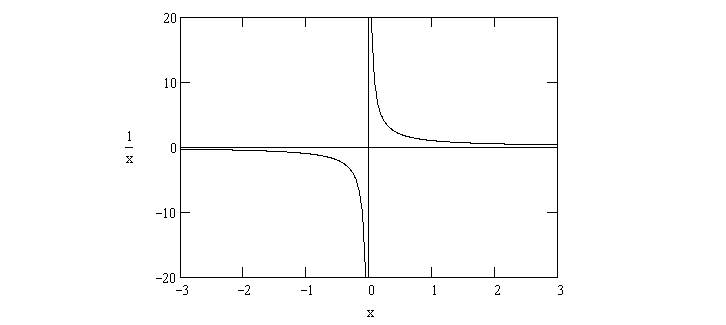Java Tutorial

# How to handle Java ArithmeticException?

Java ArithmeticException is a runtime exception that occurs when an arithmetic operation encounters an exceptional condition, such as division by zero or an integer overflow. To handle ArithmeticException in Java, you can use try-catch blocks to gracefully manage such situations and prevent the program from crashing.

## Division by Zero

public class ArithmeticExceptionExample { public static void main(String[] args) { int numerator = 10; int denominator = 0; try { int result = numerator / denominator; System.out.println("Result: " + result); } catch (ArithmeticException e) { System.out.println("Error: " + e.getMessage()); // Perform error handling or take necessary actions } } }

In this example, the program attempts to divide numerator by denominator, which is zero. This will result in an ArithmeticException. The try-catch block catches the exception and prints an error message. You can include additional error-handling logic or alternative computation within the catch block as needed.

## Integer Overflow

public class IntegerOverflowExample { public static void main(String[] args) { int maxInt = Integer.MAX_VALUE; try { int result = maxInt + 1; // This will cause integer overflow System.out.println("Result: " + result); } catch (ArithmeticException e) { System.out.println("Error: " + e.getMessage()); // Perform error handling or take necessary actions } } }

In the above example, the program attempts to add 1 to the maximum integer value (maxInt), which will cause an integer overflow, resulting in an ArithmeticException. The try-catch block catches the exception and handles it accordingly.### Conclusion

Using try-catch blocks, you can gracefully handle Java ArithmeticExceptions and implement custom error-handling logic to recover from exceptional arithmetic situations in your Java programs. This ensures that your applications maintain stability and continue to function correctly even in the face of unexpected arithmetic errors.